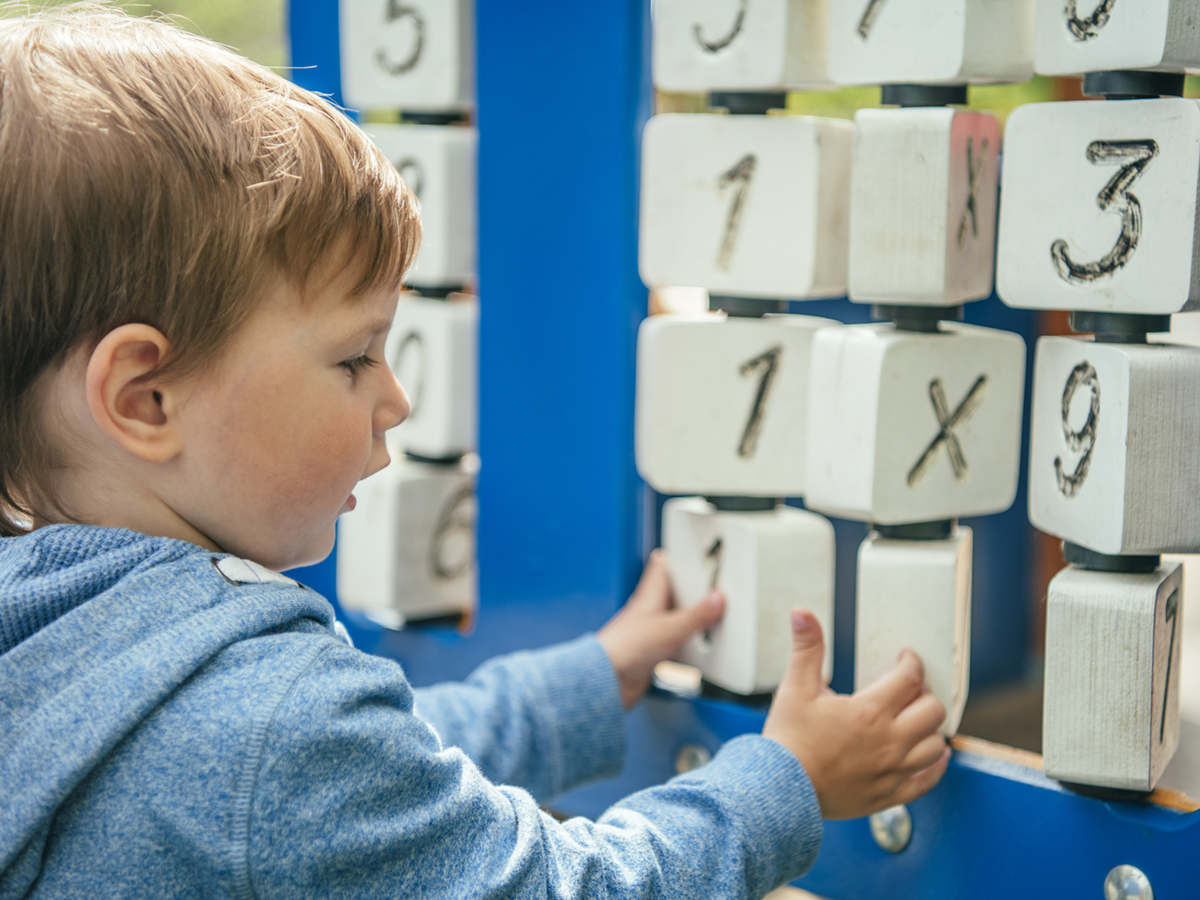# Math skills at different ages## ByAmanda MorinKids start learning math the moment they start exploring the world. Each skill — from identifying shapes to counting to finding patterns — builds on what they already know.

There are certain math milestones most kids hit at roughly the same age. But keep in mind that kids develop math skills at different rates. If kids don’t yet have all the skills listed for their age group, that’s OK.

Here’s how math skills typically develop as kids get older.

## Babies (ages 0–12 months)

• Begin to predict the sequence of events (like running water means bath time)
• Start to understand basic cause and effect (shaking a rattle makes noise)
• Begin to classify things in simple ways (some toys make noise and others don’t)
• Start to understand relative size (baby is small, parents are big)
• Begin to understand words that describe quantities (more, bigger, enough)

## Toddlers (ages 1–2 years)

• Understand that numbers mean “how many” (using fingers to show how many years old they are)
• Begin reciting numbers, but may skip some of them
• Understand words that compare or measure things (under, behind, faster)
• Match basic shapes (triangle to triangle, circle to circle)
• Explore measurement by filling and emptying containers
• Start seeing patterns in daily routines and in things like floor tiles

## Preschoolers (ages 3–4 years)

• Recognize shapes in the real world
• Start sorting things by color, shape, size, or purpose
• Compare and contrast using classifications like height, size, or gender
• Count up to at least 20 and accurately point to and count items in a group
• Understand that numerals stand for number names (5 stands for five)
• Use spatial awareness to put puzzles together
• Start predicting cause and effect (like what will happen if they drop a toy in a tub full of water)

## Kindergartners (age 5 years)

• Add by counting the fingers on one hand — 1, 2, 3, 4, 5 — and starting with 6 on the second hand
• Identify the larger of two numbers and recognize numerals up to 20
• Copy or draw symmetrical shapes
• Start using very basic maps to find a “hidden treasure”
• Begin to understand basic time concepts, like morning or days of the week
• Follow multi-step directions that use words like first and next
• Understand the meaning of words like unlikely or possible

## First and second graders

• Predict what comes next in a pattern and create own patterns
• Know the difference between two- and three-dimensional shapes and name the basic ones (cubes, cones, cylinders)
• Count to 100 by ones, twos, fives, and tens
• Write and recognize the numerals 0 to 100, and the words for numbers from one to twenty
• Do basic addition and subtraction up to 20
• Read and create a simple bar graph
• Recognize and know the value of coins

• Move from using hands-on methods to using paper and pencil to work out math problems
• Work with money
• Do addition and subtraction with regrouping (also known as borrowing)
• Understand place value well enough to solve problems with decimal points
• Know how to do multiplication and division, with help from fact families (collections of related math facts, like 3 × 4 = 12 and 4 × 3 = 12)
• Create a number sentence or equation from a word problem

## Fourth and fifth graders

• Start applying math concepts to the real world (like cutting a recipe in half)
• Practice using more than one way to solve problems
• Write and compare fractions and decimals and put them in order on a number line
• Compare numbers using > (greater than) and < (less than)
• Start two- and three-digit multiplication (like 312 × 23)
• Complete long division, with or without remainders
• Estimate and round

## Middle-schoolers

• Begin basic algebra with one unknown number (like 2 + x = 10)
• Use coordinates to locate points on a grid, also known as graphing ordered pairs
• Work with fractions, percentages, and proportions
• Work with lines, angles, types of triangles, and other basic geometric shapes
• Use formulas to solve complicated problems and to find the area, perimeter, and volume of shapes

## High-schoolers

• Understand that numbers can be represented in many ways (fractions, decimals, bases, and variables)
• Use numbers in real-life situations (like calculating a sale price or comparing student loans)
• Begin to see how math ideas build on one another
• Begin to understand that some math problems don’t have real-world solutions
• Use mathematical language to convey thoughts and solutions
• Use graphs, maps, or other representations to learn and convey information

Remember that kids develop at different paces. Some may gain some math skills later than other kids or have some that are advanced for their age.

If you're concerned about progress in math, find out why some kids have trouble with math, and next steps to take.

## About the author## About the author

Amanda Morin is the author of “The Everything Parent’s Guide to Special Education” and the former director of thought leadership at Understood. As an expert and writer, she helped build Understood from its earliest days.

## Reviewed by## Reviewed by

Brendan R. Hodnett, MAT is a special education teacher in Middletown, New Jersey, and an adjunct professor at Hunter College.